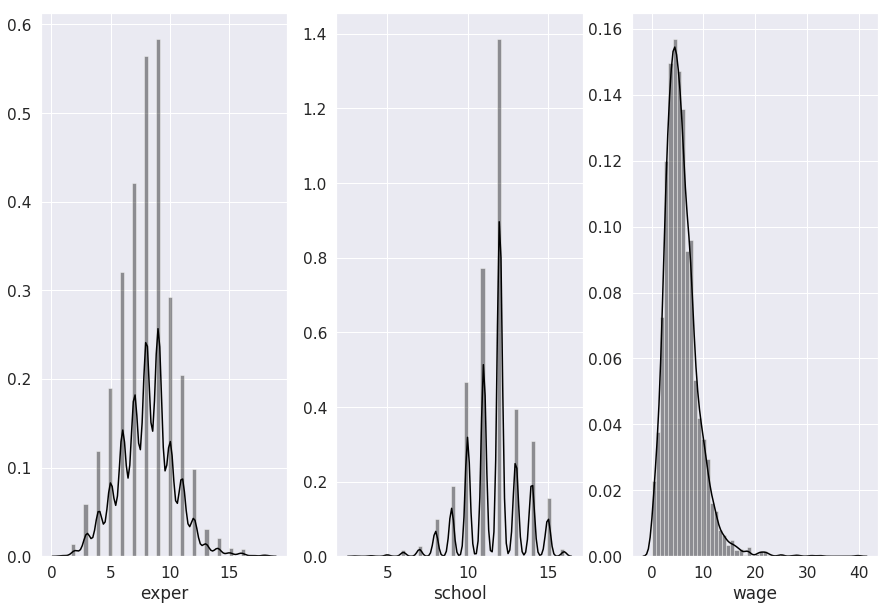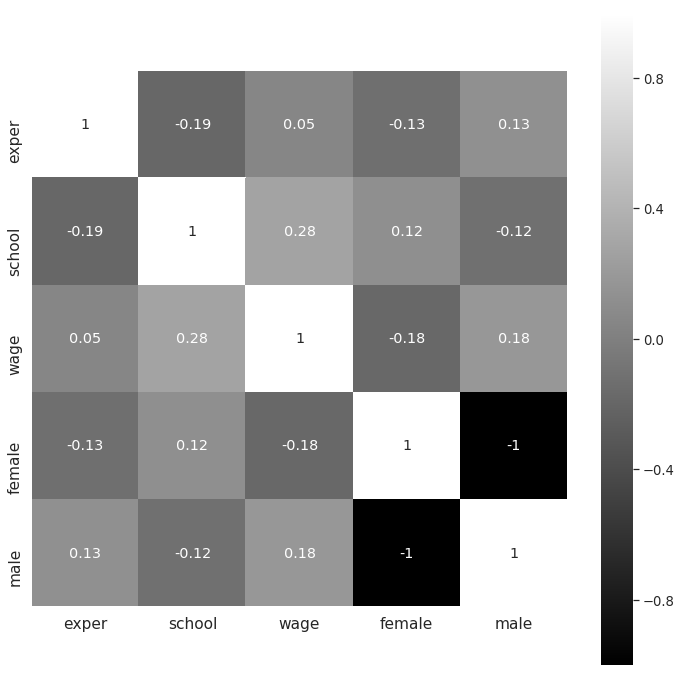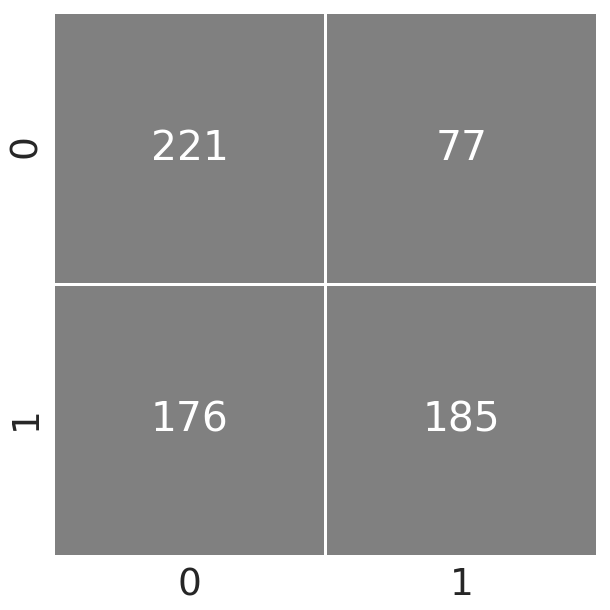# Quadratic Discriminant Analysis with Python

Quadratic discriminant analysis allows for the classifier to assess non -linear relationships. This of course something that linear discriminant analysis is not able to do. This post will go through the steps necessary to complete a qda analysis using Python. The steps that will be conducted are as follows1. Data preparation
2. Model training
3. Model testing

Our goal will be to predict the gender of examples in the “Wages1” dataset using the available independent variables.

Data Preparation

```import pandas as pd
from pydataset import data
import matplotlib.pyplot as plt
from sklearn.discriminant_analysis import QuadraticDiscriminantAnalysis as QDA
from sklearn.model_selection import train_test_split
from sklearn.metrics import classification_report
from sklearn.metrics import (confusion_matrix,accuracy_score)
import seaborn as sns
from matplotlib.colors import ListedColormap```

Next, we will load our data “Wages1” it comes from the “pydataset” library. After loading the data, we will use the .head() method to look at it briefly.We need to transform the variable ‘sex’, our dependent variable, into a dummy variable using numbers instead of text. We will use the .getdummies() method to make the dummy variables and then add them to the dataset using the .concat() method. The code for this is below.

In the code below we have the histogram for the continuous independent variables.  We are using the .distplot() method from seaborn to make the histograms.

```fig = plt.figure()
fig, axs = plt.subplots(figsize=(15, 10),ncols=3)
sns.set(font_scale=1.4)
sns.distplot(df['exper'],color='black',ax=axs)
sns.distplot(df['school'],color='black',ax=axs)
sns.distplot(df['wage'],color='black',ax=axs)```The variables look reasonable normal. Below is the proportions of the categorical dependent variable.

```round(df.groupby('sex').count()/3294,2)
Out:
exper school wage female male
sex
female 0.48 0.48 0.48 0.48 0.48
male 0.52 0.52 0.52 0.52 0.52```

About half male and half female.

We will now make the correlational matrix

```corrmat=df.corr(method='pearson')
f,ax=plt.subplots(figsize=(12,12))
sns.set(font_scale=1.2)
sns.heatmap(round(corrmat,2),
vmax=1.,square=True,
cmap="gist_gray",annot=True)```There appears to be no major problems with correlations. The last thing we will do is set up our train and test datasets.

```X=df[['exper','school','wage']]
y=df['male']
X_train,X_test,y_train,y_test=train_test_split(X,y,
test_size=.2, random_state=50)```

We can now move to model development

Model Development

To create our model we will instantiate an instance of the quadratic discriminant analysis function and use the .fit() method.

```qda_model=QDA()
qda_model.fit(X_train,y_train)```

There are some descriptive statistics that we can pull from our model. For our purposes, we will look at the group means  Below are the  group means.

 exper school wage Female 7.73 11.84 5.14 Male 8.28 11.49 6.38

You can see from the table that mean generally have more experience, higher wages, but slightly less education.

We will now use the qda_model we create to predict the classifications for the training set. This information will be used to make a confusion matrix.

```cm = confusion_matrix(y_train, y_pred)
ax= plt.subplots(figsize=(10,10))
sns.set(font_scale=3.4)
with sns.axes_style('white'):
sns.heatmap(cm, cbar=False, square=True, annot=True, fmt='g',
cmap=ListedColormap(['gray']), linewidths=2.5)```The information in the upper-left corner are the number of people who were female and correctly classified as female. The lower-right corner is for the men who were correctly classified as men. The upper-right corner is females who were classified as male. Lastly, the lower-left corner is males who were classified as females. Below is the actually accuracy of our model

```round(accuracy_score(y_train, y_pred),2)
Out: 0.6```

Sixty percent accuracy is not that great. However, we will now move to model testing.

Model Testing

Model testing involves using the .predict() method again but this time with the testing data. Below is the prediction with the confusion matrix.

``` y_pred=qda_model.predict(X_test)
cm = confusion_matrix(y_test, y_pred)
from matplotlib.colors import ListedColormap
ax= plt.subplots(figsize=(10,10))
sns.set(font_scale=3.4)
with sns.axes_style('white'):
sns.heatmap(cm, cbar=False, square=True,annot=True,fmt='g',
cmap=ListedColormap(['gray']),linewidths=2.5)```The results seem similar. Below is the accuracy.

```round(accuracy_score(y_test, y_pred),2)
Out: 0.62```

About the same, our model generalizes even though it performs somewhat poorly.

Conclusion

This post provided an explanation of how to do a quadratic discriminant analysis using python. This is just another potential tool that may be useful for the data scientist.